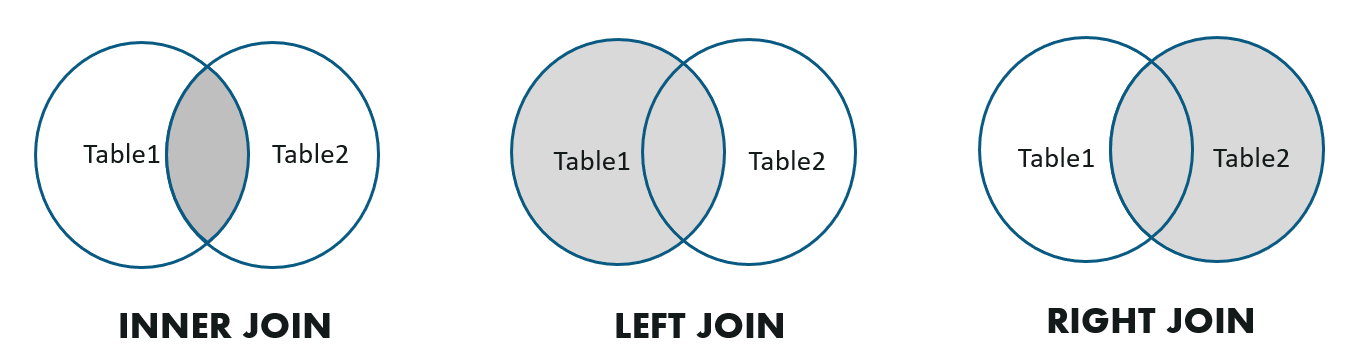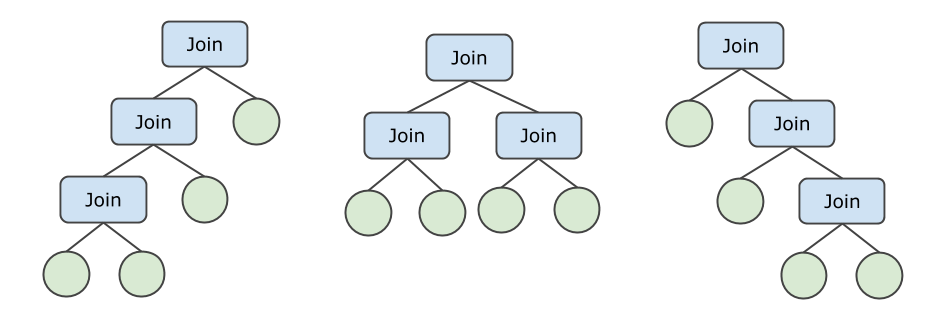## 基本概念

JOIN是SQL查询中常见的操作，逻辑上说，它的语义等价于将两张表做笛卡尔积，然后根据过滤条件保留满足条件的数据。JOIN多数情况下是依赖等值条件做的JOIN，即Equi-Join，用来根据某个特定列的值连接两张表的数据。

## JOIN类型

PolarDB-X 1.0支持Inner Join，Left Outer Join和Right Outer Join这3种常见的JOIN类型。``````/* Inner Join */
SELECT * FROM A, B WHERE A.key = B.key;
/* Left Outer Join */
SELECT * FROM A LEFT JOIN B ON A.key = B.key;
/* Right Outer Join */
SELECT * FROM A RIGHT OUTER JOIN B ON A.key = B.key;``````

``````/* Semi Join - 1 */
SELECT * FROM Emp WHERE Emp.DeptName IN (
SELECT DeptName FROM Dept
)
/* Semi Join - 2 */
SELECT * FROM Emp WHERE EXISTS (
SELECT * FROM Dept WHERE Emp.DeptName = Dept.DeptName
)
/* Anti Join - 1 */
SELECT * FROM Emp WHERE Emp.DeptName NOT IN (
SELECT DeptName FROM Dept
)
/* Anti Join - 2 */
SELECT * FROM Emp WHERE NOT EXISTS (
SELECT * FROM Dept WHERE Emp.DeptName = Dept.DeptName
)``````

## JOIN算法

Nested-Loop Join (NLJoin)

Nested-Loop Join通常用于非等值的JOIN。它的工作方式如下：

1. 拉取内表（右表，通常是数据量较小的一边）的全部数据，缓存到内存中。
2. 遍历外表数据，对于外表的每行：
• 对于每一条缓存在内存中的内表数据。
• 构造结果行，并检查是否满足JOIN条件，如果满足条件则输出。

``````> EXPLAIN SELECT * FROM partsupp, supplier WHERE ps_suppkey < s_suppkey;

NlJoin(condition="ps_suppkey < s_suppkey", type="inner")
Gather(concurrent=true)
LogicalView(tables="partsupp_[0-7]", shardCount=8, sql="SELECT * FROM `partsupp` AS `partsupp`")
Gather(concurrent=true)
LogicalView(tables="supplier_[0-7]", shardCount=8, sql="SELECT * FROM `supplier` AS `supplier`")``````

``/*+TDDL:NL_JOIN(outer_table, inner_table)*/ SELECT ...``

``/*+TDDL:NL_JOIN((outer_table_a, outer_table_b), (inner_table_c, inner_table_d))*/ SELECT ...``

Hash Join

Hash Join是等值JOIN最常用的算法之一。它的原理如下所示：

• 拉取内表（右表，通常是数据量较小的一边）的全部数据，写进内存中的哈希表。
• 遍历外表数据，对于外表的每行：
• 根据等值条件JOIN Key查询哈希表，取出0-N匹配的行（JOIN Key相同）。
• 构造结果行，并检查是否满足JOIN条件，如果满足条件则输出。

``````> EXPLAIN SELECT * FROM partsupp, supplier WHERE ps_suppkey = s_suppkey;

HashJoin(condition="ps_suppkey = s_suppkey", type="inner")
Gather(concurrent=true)
LogicalView(tables="partsupp_[0-7]", shardCount=8, sql="SELECT * FROM `partsupp` AS `partsupp`")
Gather(concurrent=true)
LogicalView(tables="supplier_[0-7]", shardCount=8, sql="SELECT * FROM `supplier` AS `supplier`")``````

Hash Join常出现在JOIN数据量较大的复杂查询、且无法通过索引Lookup来改善，这种情况下Hash Join是最优的选择。例如上面的例子中，partsupp表和supplier表均为全表扫描，数据量较大，适合使用HashJoin。

``/*+TDDL:HASH_JOIN(table_outer, table_inner)*/ SELECT ...``

Lookup Join (BKAJoin)

Lookup Join是另一种常用的等值JOIN算法，常用于数据量较小的情况。它的原理如下：

1. 遍历外表（左表，通常是数据量较小的一边）数据，对于外表中的每批（例如1000行）数据。
2. 将这一批数据的JOIN Key拼成一个`IN (....)`条件，加到内表的查询中。
3. 执行内表查询，得到JOIN匹配的行。
4. 借助哈希表，为外表的每行找到匹配的内表行，组合并输出。

``````> EXPLAIN SELECT * FROM partsupp, supplier WHERE ps_suppkey = s_suppkey AND ps_partkey = 123;

BKAJoin(condition="ps_suppkey = s_suppkey", type="inner")
LogicalView(tables="partsupp_3", sql="SELECT * FROM `partsupp` AS `partsupp` WHERE (`ps_partkey` = ?)")
Gather(concurrent=true)
LogicalView(tables="supplier_[0-7]", shardCount=8, sql="SELECT * FROM `supplier` AS `supplier` WHERE (`s_suppkey` IN ('?'))")``````

Lookup Join通常用于外表数据量较小的情况，例如上面的例子中，左表partsupp由于存在`ps_partkey = 123`的过滤条件，仅有几行数据。此外，右表的`s_suppkey IN ( ... )`查询命中了主键索引，这也使得Lookup Join的查询代价进一步降低。

``/*+TDDL:BKA_JOIN(table_outer, table_inner)*/ SELECT ...``

Sort-Merge Join

Sort-Merge Join是另一种等值JOIN算法，它依赖左右两边输入的顺序，必须按JOIN Key排序。它的原理如下：

1. 开始Sort-Merge Join之前，输入端必须排序（借助MergeSort或MemSort）。
2. 比较当前左右表输入的行，并按以下方式操作，不断消费左右两边的输入：
• 如果左表的JOIN Key较小，则消费左表的下一条数据。
• 如果右表的JOIN Key较小，则消费右表的下一条数据。
• 如果左右表JOIN Key相等，说明获得了1条或多条匹配，检查是否满足JOIN条件并输出。

``````> EXPLAIN SELECT * FROM partsupp, supplier WHERE ps_suppkey = s_suppkey ORDER BY s_suppkey;

SortMergeJoin(condition="ps_suppkey = s_suppkey", type="inner")
MergeSort(sort="ps_suppkey ASC")
LogicalView(tables="QIMU_0000_GROUP,QIMU_0001_GROUP.partsupp_[0-7]", shardCount=8, sql="SELECT * FROM `partsupp` AS `partsupp` ORDER BY `ps_suppkey`")
MergeSort(sort="s_suppkey ASC")
LogicalView(tables="QIMU_0000_GROUP,QIMU_0001_GROUP.supplier_[0-7]", shardCount=8, sql="SELECT * FROM `supplier` AS `supplier` ORDER BY `s_suppkey`")``````

Sort-Merge Join由于需要额外的排序步骤，通常Sort-Merge Join并不是最优的。但是，某些情况下客户端查询恰好也需要按JOIN Key排序（上面的例子），这时候使用Sort-Merge Join是较优的选择。

``/*+TDDL:SORT_MERGE_JOIN(table_a, table_b)*/ SELECT ...``

## JOIN顺序• 当（未下推的）N较小时，采取Bushy枚举策略，会在所有JOIN顺序中选出最优的计划。
• 当（未下推的）表的数量较多时，采取Zig-Zag（锯齿状）或Left-Deep（左深树）的枚举策略，选出最优的Zig-Zag或Left-Deep执行计划，以减少枚举的次数和代价。

PolarDB-X 1.0使用基于代价的优化器（Cost-based Optimizer，CBO）选择出总代价最低的JOIN 顺序。详情参见查询优化器介绍

## 子查询

``````/* 例子：非关联子查询 */
SELECT * FROM lineitem WHERE l_partkey IN (SELECT p_partkey FROM part);

/* 例子：关联子查询（l_suppkey 是关联项） */
SELECT * FROM lineitem WHERE l_partkey IN (SELECT ps_partkey FROM partsupp WHERE ps_suppkey = l_suppkey);``````

PolarDB-X 1.0子查询支持绝大多数的子查询写法，具体参见SQL使用限制

``````> EXPLAIN SELECT p_partkey, (
SELECT COUNT(ps_partkey) FROM partsupp WHERE ps_suppkey = p_partkey
) supplier_count FROM part;
Project(p_partkey="p_partkey", supplier_count="CASE(IS NULL(\$10), 0, \$9)", cor=[\$cor0])
HashJoin(condition="p_partkey = ps_suppkey", type="left")
Gather(concurrent=true)
LogicalView(tables="part_[0-7]", shardCount=8, sql="SELECT * FROM `part` AS `part`")
Project(count(ps_partkey)="count(ps_partkey)", ps_suppkey="ps_suppkey", count(ps_partkey)2="count(ps_partkey)")
HashAgg(group="ps_suppkey", count(ps_partkey)="SUM(count(ps_partkey))")
Gather(concurrent=true)
LogicalView(tables="partsupp_[0-7]", shardCount=8, sql="SELECT `ps_suppkey`, COUNT(`ps_partkey`) AS `count(ps_partkey)` FROM `partsupp` AS `partsupp` GROUP BY `ps_suppkey`")``````
``````> EXPLAIN SELECT p_partkey, (
SELECT COUNT(ps_partkey) FROM partsupp WHERE ps_suppkey = p_partkey
) supplier_count FROM part;

Project(p_partkey="p_partkey", supplier_count="CASE(IS NULL(\$10), 0, \$9)", cor=[\$cor0])
HashJoin(condition="p_partkey = ps_suppkey", type="left")
Gather(concurrent=true)
LogicalView(tables="part_[0-7]", shardCount=8, sql="SELECT * FROM `part` AS `part`")
Project(count(ps_partkey)="count(ps_partkey)", ps_suppkey="ps_suppkey", count(ps_partkey)2="count(ps_partkey)")
HashAgg(group="ps_suppkey", count(ps_partkey)="SUM(count(ps_partkey))")
Gather(concurrent=true)
LogicalView(tables="partsupp_[0-7]", shardCount=8, sql="SELECT `ps_suppkey`, COUNT(`ps_partkey`) AS `count(ps_partkey)` FROM `partsupp` AS `partsupp` GROUP BY `ps_suppkey`")``````

``````> EXPLAIN SELECT * FROM lineitem WHERE l_partkey IN (SELECT ps_partkey FROM partsupp WHERE ps_suppkey = l_suppkey) OR l_partkey IS NOT

Filter(condition="IS(in,[\$1]) OR l_partkey < ?0")
Gather(concurrent=true)
LogicalView(tables="QIMU_0000_GROUP,QIMU_0001_GROUP.lineitem_[0-7]", shardCount=8, sql="SELECT * FROM `lineitem` AS `lineitem`")

>> individual correlate subquery : 29612489
Gather(concurrent=true)
LogicalView(tables="QIMU_0000_GROUP,QIMU_0001_GROUP.partsupp_[0-7]", shardCount=8, sql="SELECT * FROM (SELECT `ps_partkey` FROM `partsupp` AS `partsupp` WHERE (`ps_suppkey` = `l_suppkey`)) AS `t0` WHERE (((`l_partkey` = `ps_partkey`) OR (`l_partkey` IS NULL)) OR (`ps_partkey` IS NULL))")``````